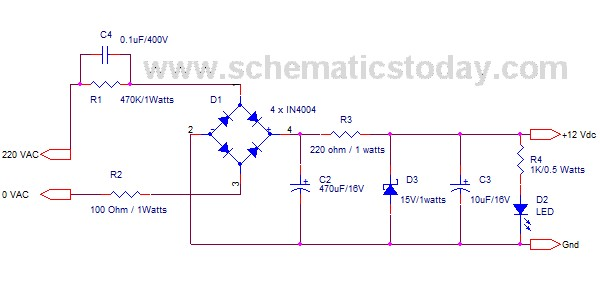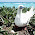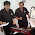## Wednesday, May 6, 2015

### Circuit Schematic 220 VAC to 12 VDC, 100mA Transformerless Power Supply

Eltronicschool. - One of the step download power supply is using transformerless with many kinds of output power. In here we will show you one of circuit schematic transformerless power supply from 220VAC to 12VDC with output current more than 100mA like in figure 1 below.

Circuit SchematicCircuit Schematic 220 VAC to 12 VDC, 100mA Transformerless Power Supply (Source: www.Schematicstoday.com)
Component Parts
• R1 = 470K / 1 Watts
• R2 = 100 / 1 Watts
• R3 = 220 / 1 Watts
• R4 = 1K / 0.5 Watts
• C4 = 0.1uF/ 400V
• D1 = IN4004
• C2 = 470uF / 16V
• D3 = Zener Diode 15V / 1 Watts
• C3 = 10uF/16V
• D2 = LED

Description

Circuit schematic like in figure 1 above is circuit schematic 220VAC to 12VDC, 100mA transformerless power supply. According the description from schematicstoday blog that low current applications like microcontroller can be supply with this transformerless power supply. C4 is used to rate AC capacitor that reduces high volt AC 220V. To rectify AC to DC in this schematic used D1 as bridge rectifier diodes. Because of the ripple after rectified, in here need C2 to remove ripples. To remove stored current in AC when power is off, it is used R3. And to get regulated DC in the output, zener diode D3 be used with C3 to reduce ripple again after regulated. R2 limits inrush current to LED D2. From this combination output of this circuit schematic is more than +12VDC, 100mA.

Thank you for your coming here in www.eltronicschool.com site, we hope the article above will help you to know more about your an electronic circuit design and software in this time, etc. Please comment here when you want to share and other. Thank you.

1.Your article is helped me to understand the transformer less 220VAC to 12VDC converter. Thank you for that.

I tried to experiment with that diagram. I used 225j 400v x rated capacitor instead of 0.1uF 400v and the remaining components were same to your circuit's components. But when i connected the circuit with 220v AC then there was a little spark and my 100 ohm/1 watt resistor was damaged. Can you suggest me what can I do now?

2.When you change the capacitor (C4), it mean that it will change the impedance (Z) of C4 and R1. with C4 = 0.1uF and R1 = 470K, it mean that Z = 31774.3 ohm (note: f=50Hz). When you change C4 = 0.22uf (225J) with R = 470K it make Z = 14469.3 ohm (note: f=50hz). It mean that the impedance (Z) smaller that make the current larger and can make the Resistor as the resistance damage. So, you can use the C with 0.1uF or Smaller that make the Z same or bigger.